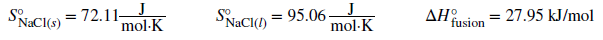Chemistry Practice Problems Entropy Practice Problems Solution: By calculating ΔSuniv at each temperature, determi...

⚠️Our tutors found the solution shown to be helpful for the problem you're searching for. We don't have the exact solution yet.

# Solution: By calculating ΔSuniv at each temperature, determine if the melting of 1 mole of NaCl(s) is spontaneous at 500 °C and at 700 °C.What assumptions are made about the thermodynamic information (entropy and enthalpy values) used to solve this problem?

###### Problem

By calculating ΔSuniv at each temperature, determine if the melting of 1 mole of NaCl(s) is spontaneous at 500 °C and at 700 °C.What assumptions are made about the thermodynamic information (entropy and enthalpy values) used to solve this problem?

Entropy

Entropy

#### Q. Use the standard entropy data in Appendix G to determine the change in entropy for each of the reactions listed below. All the processes occur at the ...

Solved • Thu Nov 29 2018 10:22:33 GMT-0500 (EST)

Entropy

#### Q. Using the relevant S°298 values listed in Appendix G, calculate ΔS°298 for the following change:(a) N2(g) + 3H2(g) ⟶ 2NH3(g)

Solved • Thu Nov 29 2018 09:55:04 GMT-0500 (EST)

Entropy

#### Q. “Thermite” reactions have been used for welding metal parts such as railway rails and in metal refining. One such thermite reaction is Fe2 O3(s) + 2Al...

Solved • Thu Nov 29 2018 09:45:18 GMT-0500 (EST)

Entropy

#### Q. Determine the entropy change for the combustion of gaseous propane, C 3H8, under the standard conditions to give gaseous carbon dioxide and water.

Solved • Thu Nov 29 2018 09:42:42 GMT-0500 (EST)